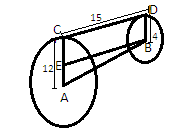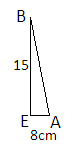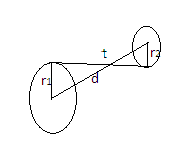QuestionAnswers

# The length of common tangent to the circle of radii $12$cm and $4$cm is $15$ cm. The distance between their centers is $17$cm.A) True B) FalseHint: We can solve the problem by using pythagoras theorem by making figure on given conditions or we can also solve using the formula ${\text{d = }}\sqrt {{{\text{t}}^2} + {{\left( {{{\text{r}}_1} - {{\text{r}}_2}} \right)}^2}}$ where d is the distance between the centers of two circles with radii ${{\text{r}}_1}$ and ${{\text{r}}_2}$, and t is the length of the common tangent. On putting the given values, you’ll get the answer.

Given, the length of common tangent to the circles = $15$cm and the radii of the two circles are $12$cm and $4$cm. We have to find the distance between their centers. Let A and B be the centers of the two circles respectively and CD be the common tangent to the circles. Let us draw EB $\parallel$CD to make rectangle BDCE where CD=EB=$15$cm and EC=BD= $4$cmNow from the figure, AE=AC-CE
$\Rightarrow {\text{AE = 12 - 4 = 8}}$ cmNow In the triangle BEA, We know EB and AE but we have to find AB.
Let AB=x cm. Since ∆ BEA is a right-angled triangle, we can use the Pythagoras theorem to find AB. According to the Pythagoras theorem, “In a right-angled triangle, the square of the hypotenuse side is equal to the sum of squares of the other two sides.”It can be written as-
$\Rightarrow$ ${{\text{H}}^2} = {{\text{P}}^2}{\text{ + }}{{\text{B}}^2}$
Where H is the hypotenuse, P is the perpendicular and B is the base of the triangle.
In triangle BEA, H=AB, P=EB and B=AE
Then on using the formula, we get
$\Rightarrow$ ${\left( {{\text{AE}}} \right)^2} + {\left( {{\text{EB}}} \right)^2} = {\text{A}}{{\text{B}}^2}$
$\Rightarrow {{\text{x}}^2} = {\left( 8 \right)^2} + {\left( {15} \right)^2} = 64 + 225 = 289 \\ \Rightarrow {\text{x}} = \sqrt {289} = 17 \\$
AB=$17$ cm= the distance between the centers of the two circles. So the statement is true.
Hence the correct answer is ‘A’.
Note:: We can also use the formula ${\text{d = }}\sqrt {{{\text{t}}^2} + {{\left( {{{\text{r}}_1} - {{\text{r}}_2}} \right)}^2}}$ where d is the distance between the centers of two circles with radii ${{\text{r}}_1}$ and${{\text{r}}_2}$, and t is the length of the common tangent and we’ll get the same answer. This formula is used when the circles have a direct common tangent. On putting the given values we get,
$\Rightarrow$ d=$\sqrt {{{15}^2} + {{\left( {12 - 4} \right)}^2}} = \sqrt {225 + {8^2}} = \sqrt {225 + 64}$
$\Rightarrow$ d=$\sqrt {289} = 17$ cm
But If the circles have transverse common tangent (see the figure)-Then we use formula for distance between their centers ${\text{d = }}\sqrt {{{\text{t}}^2} + {{\left( {{{\text{r}}_1}{\text{ + }}{{\text{r}}_2}} \right)}^2}}$ where the notations have same meaning.
View Notes
Area of a Sector of a Circle FormulaMeasurement of Length DistanceRelation Between the Length of a Given Wire and Tension for Constant Frequency Using SonometerTo Find the Weight of a Given Body Using Parallelogram Law of VectorsTo Determine the Mass of Two Different Objects Using a Beam BalanceTangent FormulaTo Measure the Volume of an Irregular Lamina Using Screw GaugeThe Making of a ScientistChanging the Period of a PendulumThe Idea of TimeCBSE Class 8 Science Reaching The Age of Adolescence WorksheetsImportant Questions for CBSE Class 6 English A Pact with The Sun Chapter 9 - What Happened to The ReptilesImportant Questions for CBSE Class 6 Social Science The Earth Our Habitat Chapter 3 - Motions of the EarthImportant Questions for CBSE Class 12 Micro Economics Chapter 4 - Theory of the Firm under Perfect CompetitionImportant Questions for CBSE Class 11 English Snapshots Chapter 1 - The Summer of the Beautiful White HorseImportant Questions for CBSE Class 9 Science Chapter 4 - Structure of The AtomImportant Questions for CBSE Class 6 Social Science The Earth Our Habitat Chapter 5 - Major Domains Of The EarthImportant Questions for CBSE Class 7 English Honeycomb Chapter 10 - The Story of CricketImportant Questions for CBSE Class 6 Social Science The Earth Our Habitat Chapter 6 - Major Landforms Of The EarthImportant Questions for CBSE Class 8 English Honeydew Chapter 3 - Glimpses of the PastPrevious Year Question Paper of CBSE Class 10 EnglishCBSE Class 12 Maths Question Paper 2020Maths Question Paper for CBSE Class 12 - 2013Previous Year Question Paper for CBSE Class 12 Maths - 2014CBSE Previous Year Question Papers Class 12 Maths with SolutionsMaths Question Paper for CBSE Class 12 - 2016 Set 1 CMaths Question Paper for CBSE Class 12 - 2016 Set 1 EMaths Question Paper for CBSE Class 12 - 2016 Set 1 SCBSE Class 12 Maths Question Paper 2018 with Solutions - Free PDFCBSE Class 12 Maths Question Paper 2015 with Solutions - Free PDFRD Sharma Class 11 Maths Solutions Chapter 24 - The CircleNCERT Solutions for Class 12 English Vistas Chapter 3 - Journey to the end of the EarthTextbooks Solutions for CBSE & ICSE Board of Class 6 to 12 Maths & ScienceRD Sharma Class 11 Solutions Chapter 24 - The Circle (Ex 24.2) Exercise 24.2RD Sharma Class 11 Solutions Chapter 24 - The Circle (Ex 24.1) Exercise 24.1RD Sharma Class 11 Solutions Chapter 24 - The Circle (Ex 24.3) Exercise 24.3RD Sharma Class 7 Solutions Chapter 20 - Mensuration I (Area of Circle) (Ex 20.2) Exercise 20.2RD Sharma Class 7 Solutions Chapter 20 - Mensuration I (Area of Circle) (Ex 20.1) Exercise 20.1NCERT Solutions for Class 12 English Vistas Chapter 6 - On the Face of itReaching The Age of Adolescence NCERT Solutions - Class 8 Science